Type
Solution Manual
Book Title
Fundamentals of Investments: Valuation and Management 8th Edition
ISBN 13
978-1259720697

### 978-1259720697 Chapter 17 Solution Manual

January 2, 2020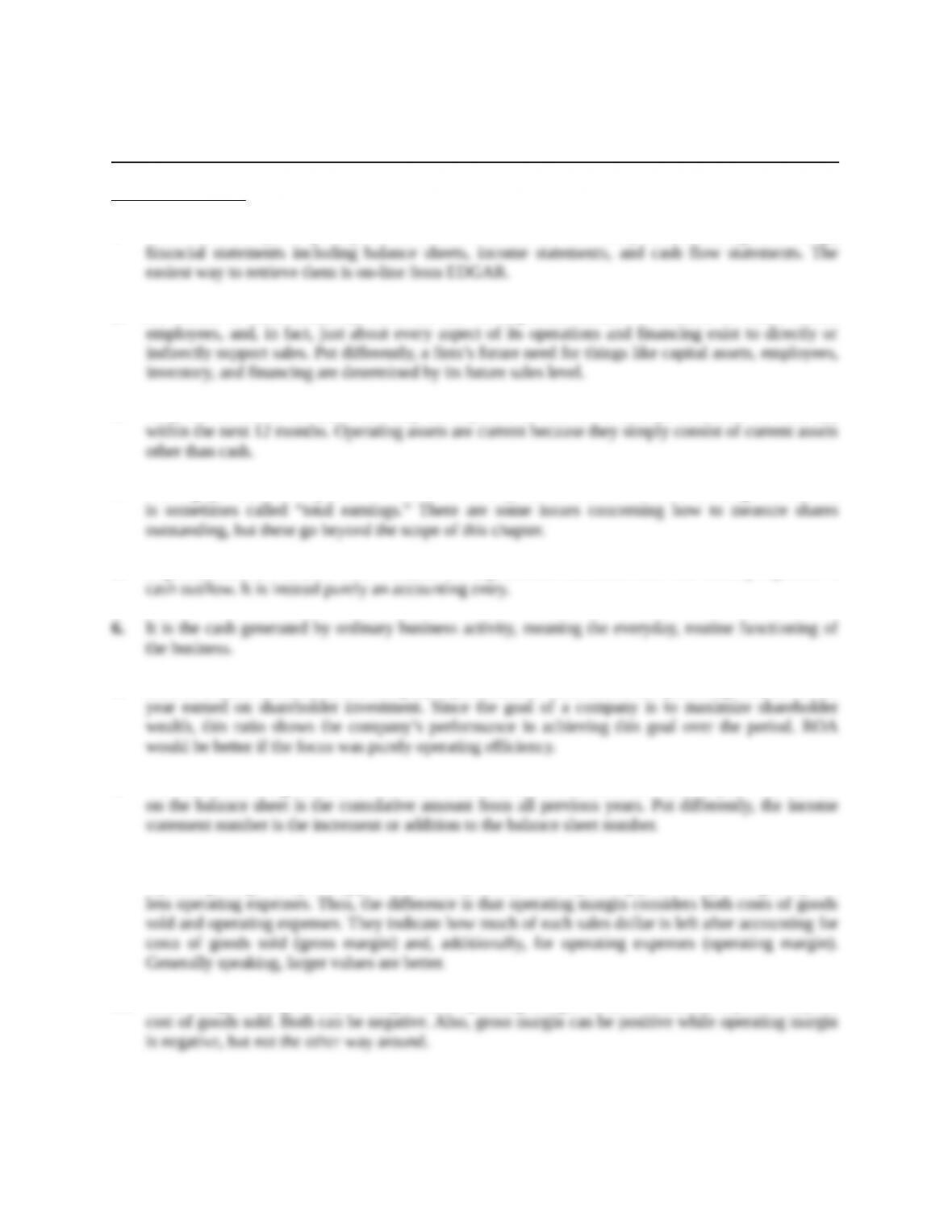Chapter 17
Projecting Cash Flow and Earnings
Concept Questions
1. The 10K and 10Q are reports public firms must file with the SEC. They contain, among other things,
2. The reason is that, ultimately, sales are the driving force behind a business. A firm’s assets,
3. They are current in the sense that they are expected to convert to cash (or otherwise be used up)
4. Earnings per share are equal to net income divided by the number of shares outstanding. Net income
5. Depreciation is a “noncash item” because the depreciation deduction does not literally represent a
7. ROE is a better measure of the company’s performance. ROE shows the percentage return for the
8. The retained earnings number on the income statement is the amount retained that year. The number
9. Gross margin is gross profit divided by sales, where gross profit is sales less cost of goods sold.
Operating margin is operating profit divided by sales, where operating profit is equal to gross profit
10. Gross margin will generally be larger since operating margin deducts additional expenses beyond
Education.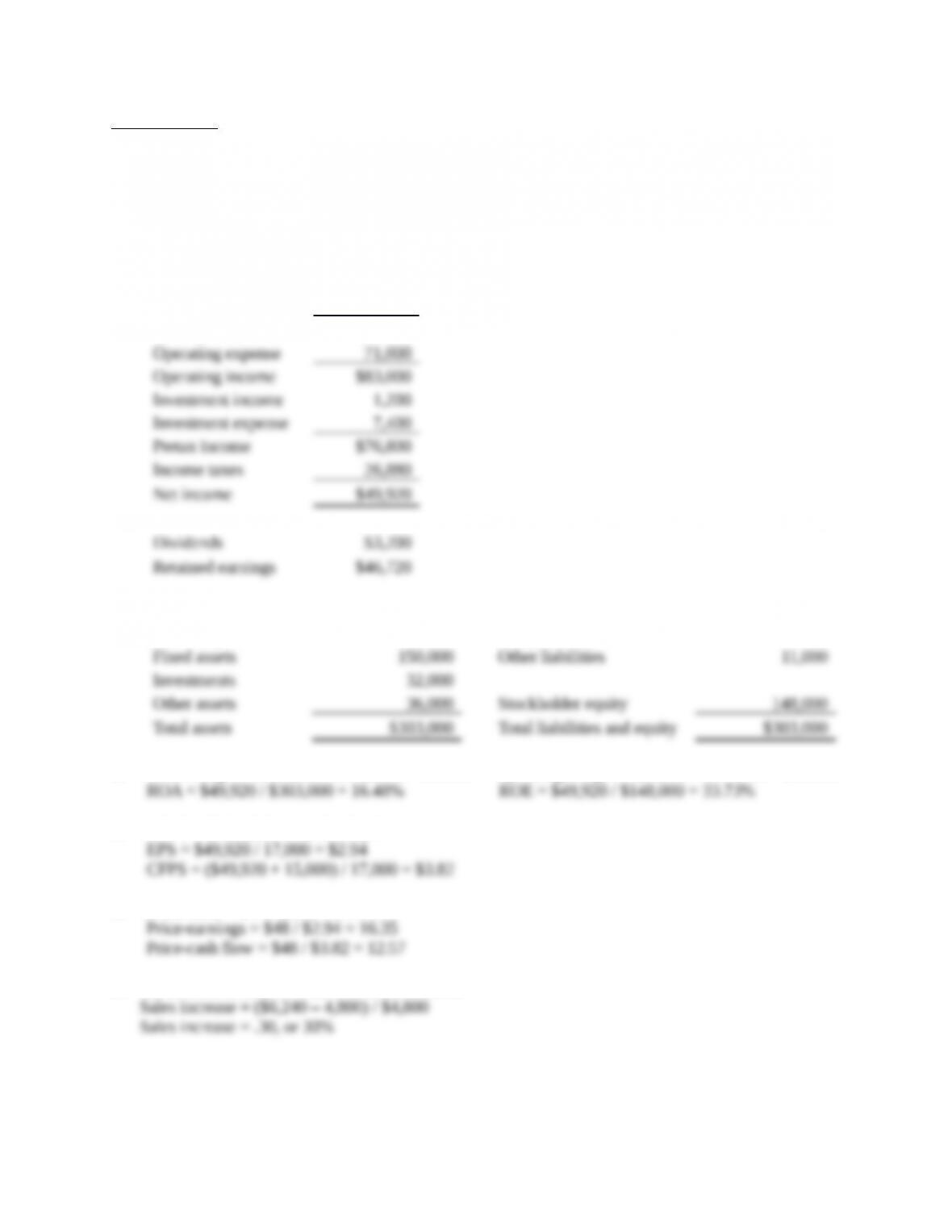Core Questions
NOTE: All end of chapter problems were solved using a spreadsheet. Many problems require multiple
steps. Due to space and readability constraints, when these intermediate steps are included in this
solutions manual, rounding may appear to have occurred. However, the final answer for each problem is
found without rounding during any step in the problem.
1. Sales \$318,000
Cost of goods sold 164,000
Gross profit \$154,000
2. Cash \$21,000 Current liabilities \$42,000
Operating assets 64,000 Long-term debt 102,000
3. Gross margin = \$154,000 / \$318,000 = 48.43% Operating margin = \$83,000 / \$318,000 = 26.10%
4. BVPS = \$148,000 / 17,000 = \$8.71
5. Price-book = \$48 / \$8.71 = 5.51
6. An increase of sales to \$6,240 is an increase of:
Education.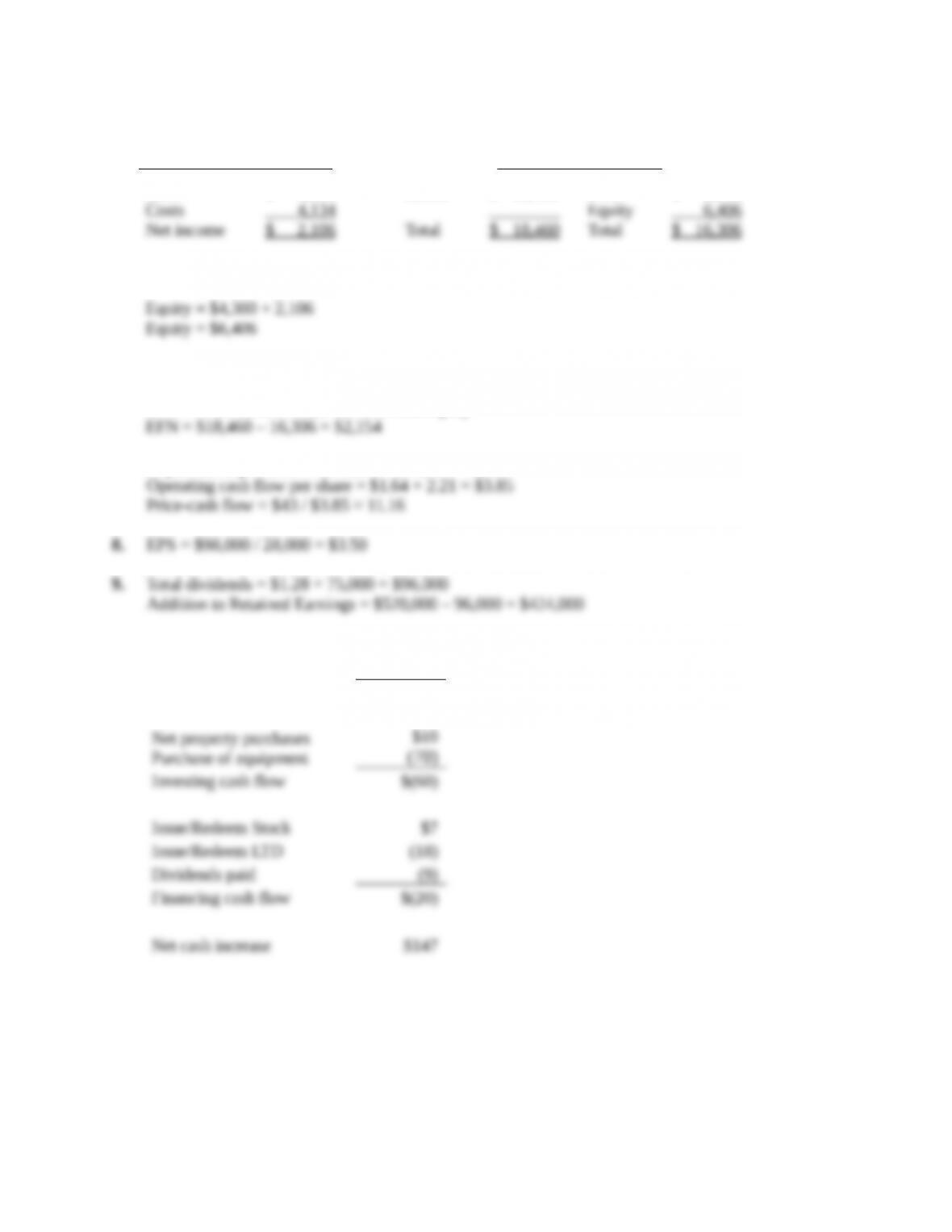Assuming costs and assets increase proportionally, the pro forma financial statements will look like
this:
Pro forma income statement Pro forma balance sheet
Sales \$ 6,240 Assets \$ 18,460 Debt \$ 9,900
If no dividends are paid, the equity account will increase by the net income, so:
So the EFN is:
EFN = Total assets – Total liabilities and equity
7. Depreciation per share = \$310,000 / 140,000 = \$2.21
10. Net income \$175
Dep and amort. 52
Operating cash flow \$227
Education.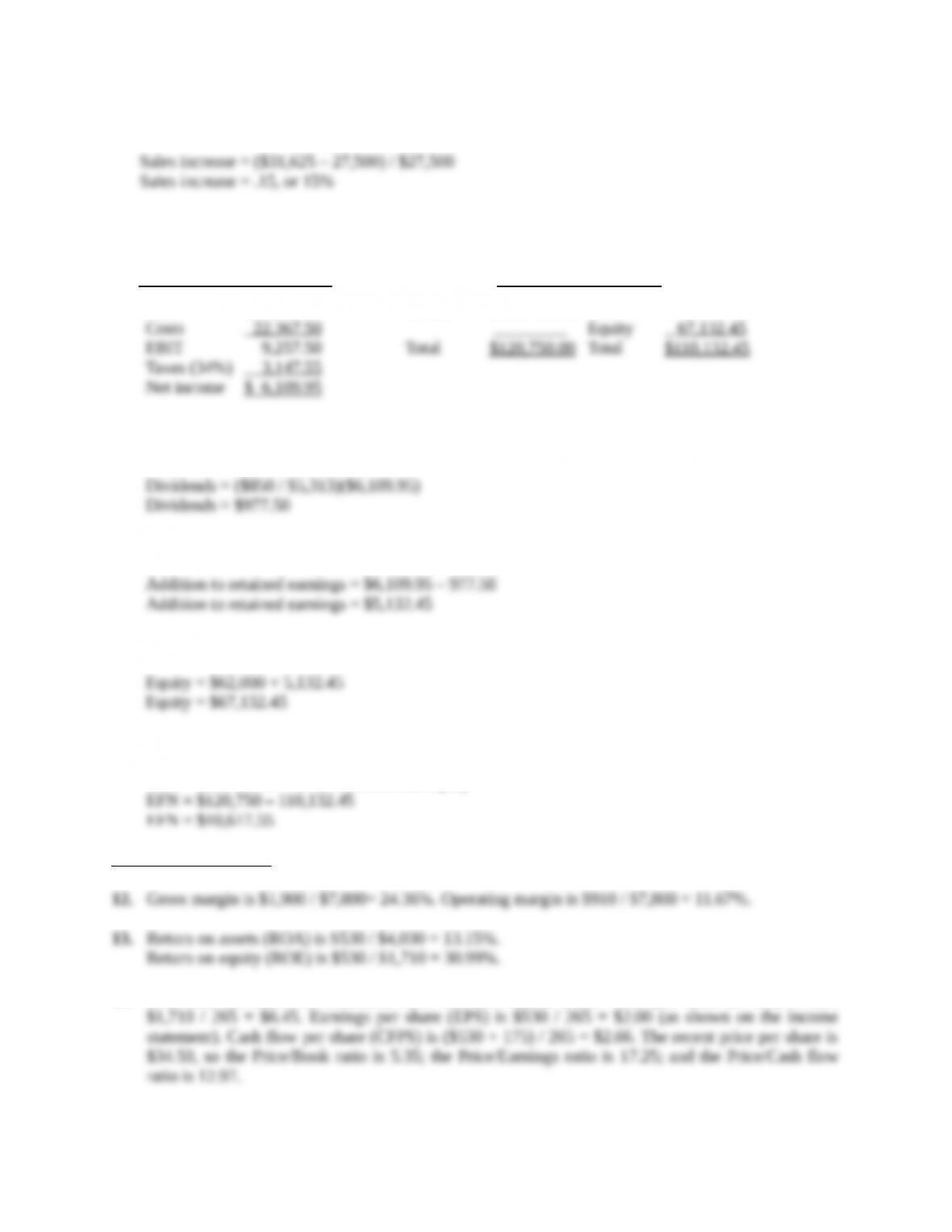11. An increase of sales to \$31,625 is an increase of:
Assuming costs and assets increase proportionally, the pro forma financial statements will look like
this:
Pro forma income statement Pro forma balance sheet
Sales \$31,625.00 Assets \$120,750.00 Debt \$ 43,000.00
The payout ratio is constant, so the dividends paid this year is the payout ratio from last year times
net income, or:
The addition to retained earnings is:
And the new equity balance is:
So the EFN is:
EFN = Total assets – Total liabilities and equity
Intermediate Questions
14. Note that, measured in thousands, there are 265 shares. Book value per share (BVPS) is thus
Education.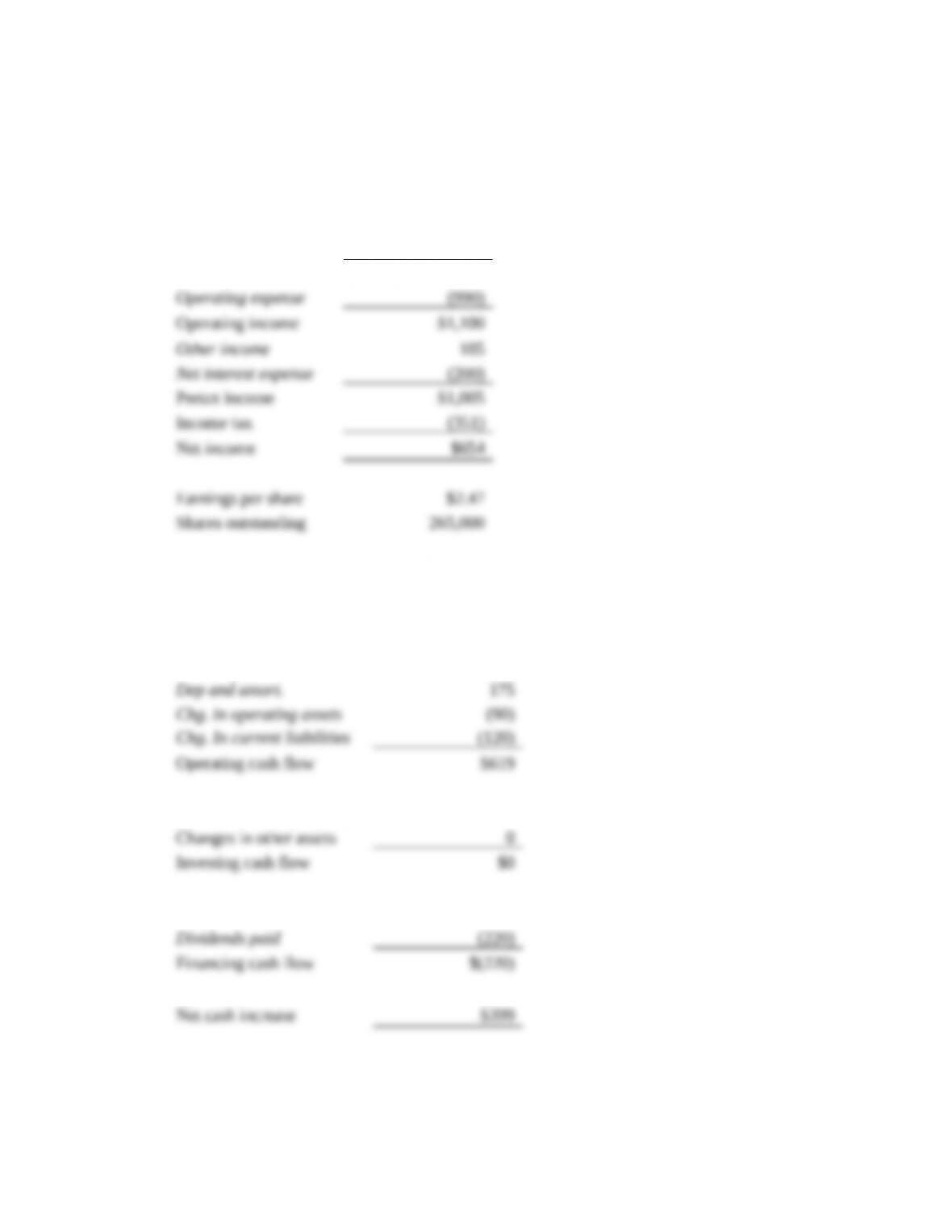15. With a 10% sales increase, sales will rise to \$8,580. A constant gross margin is assumed, implying
that Cost of Goods Sold will also increase by 10%. A constant tax rate is used. Items in italics are
carried over unchanged. The pro forma income statement follows
Kiwi Fruit Company Pro Forma Income Statement
Net sales \$8,580
Cost of goods sold (6,490)
Gross profit \$2,090
Next, we prepare the cash flow statement. Notice that we pick up the \$654 net income from the pro
forma income statement. Items in italics are carried over unchanged. By assumption, no investments
occur, and no long-term debt is issued or redeemed.
Kiwi Fruit Company Pro Forma Cash Flow Statement
Net income \$654
Issue/Redeem LTD \$0
Education.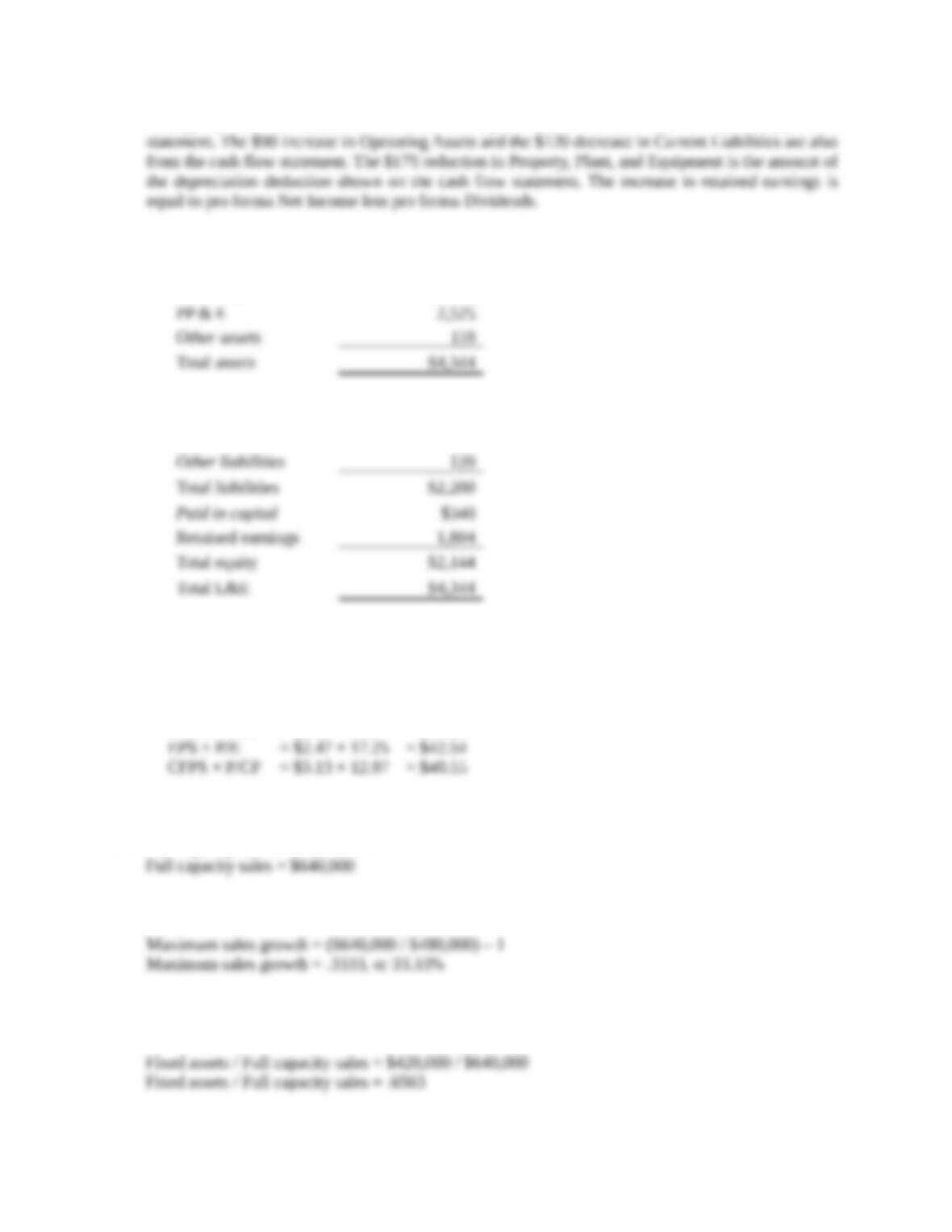Finally, we have the balance sheet. Cash rises by the \$399. Net cash flow is from the cash flow
Kiwi Fruit Company Pro Forma Balance Sheet
Cash and equiv. \$969
Operating assets 740
Current liabilities \$800
Long-term debt 1,280
We should note that the assumptions made here could vary, so good analysts may (and will) come up
with different values.
16. Using the benchmarks from question 14, projected stock prices are:
BVPS × P/B = \$8.09 × 5.35 = \$43.25
Thus, projected prices assuming a 10% sales increase are in the \$40.55 – \$43.25 range.
17. Full capacity sales = \$480,000 / .75
The maximum sales growth is the full capacity sales divided by the current sales, so:
18. To find the new level of fixed assets, we need to find the current percentage of fixed assets to full
capacity sales. Doing so, we find:
Education.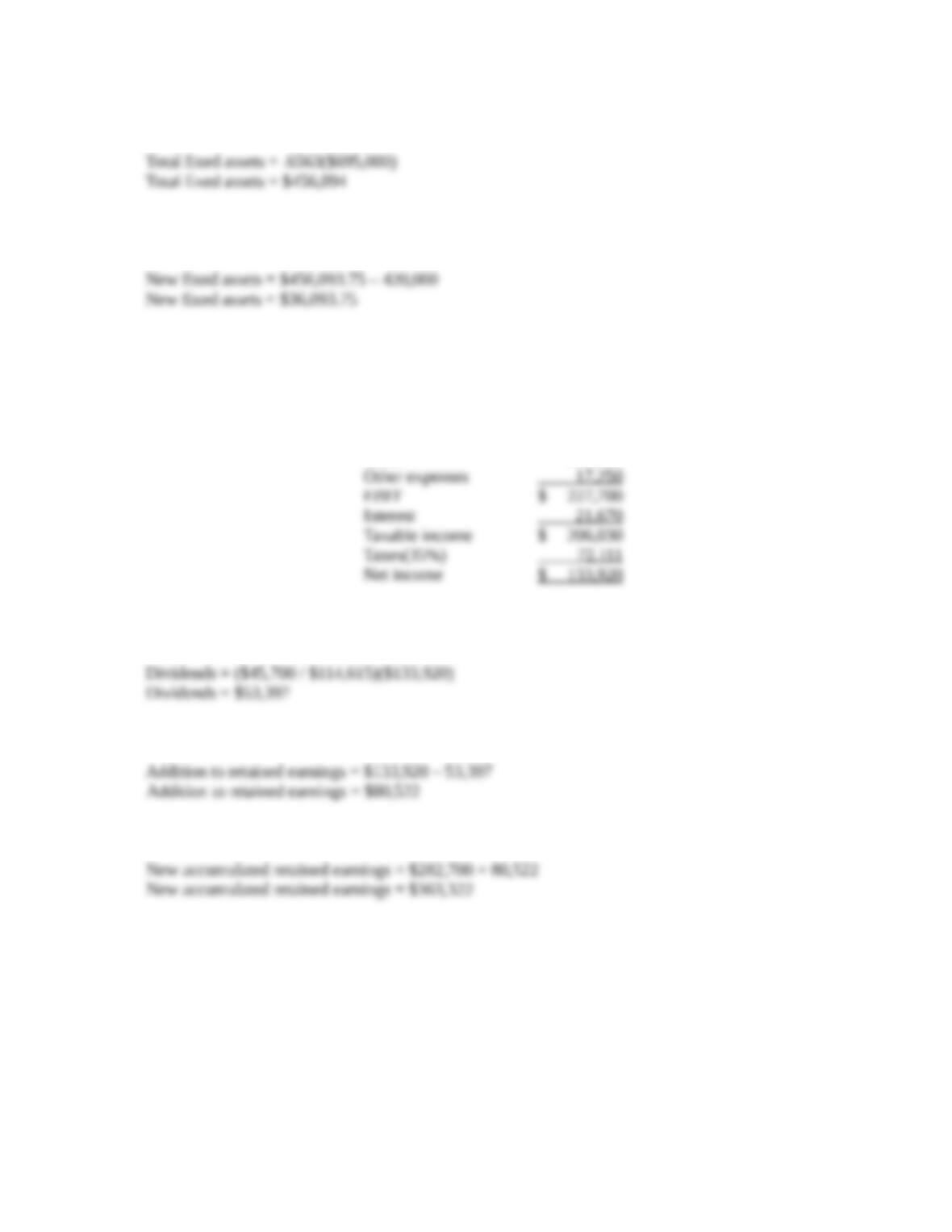Next, we calculate the total dollar amount of fixed assets needed at the new sales figure.
The new fixed assets necessary is the total fixed assets at the new sales figure minus the current level
of fixed assets.
19. Assuming costs vary with sales and a 15 percent increase in sales, the pro forma income statement
will look like this:
MOOSE TOURS INC.
Pro Forma Income Statement
Sales \$ 1,144,250
Costs 899,300
The payout ratio is constant, so the dividends paid this year is the payout ratio from last year times
net income, or:
And the addition to retained earnings will be:
The new accumulated retained earnings on the pro forma balance sheet will be:
Education.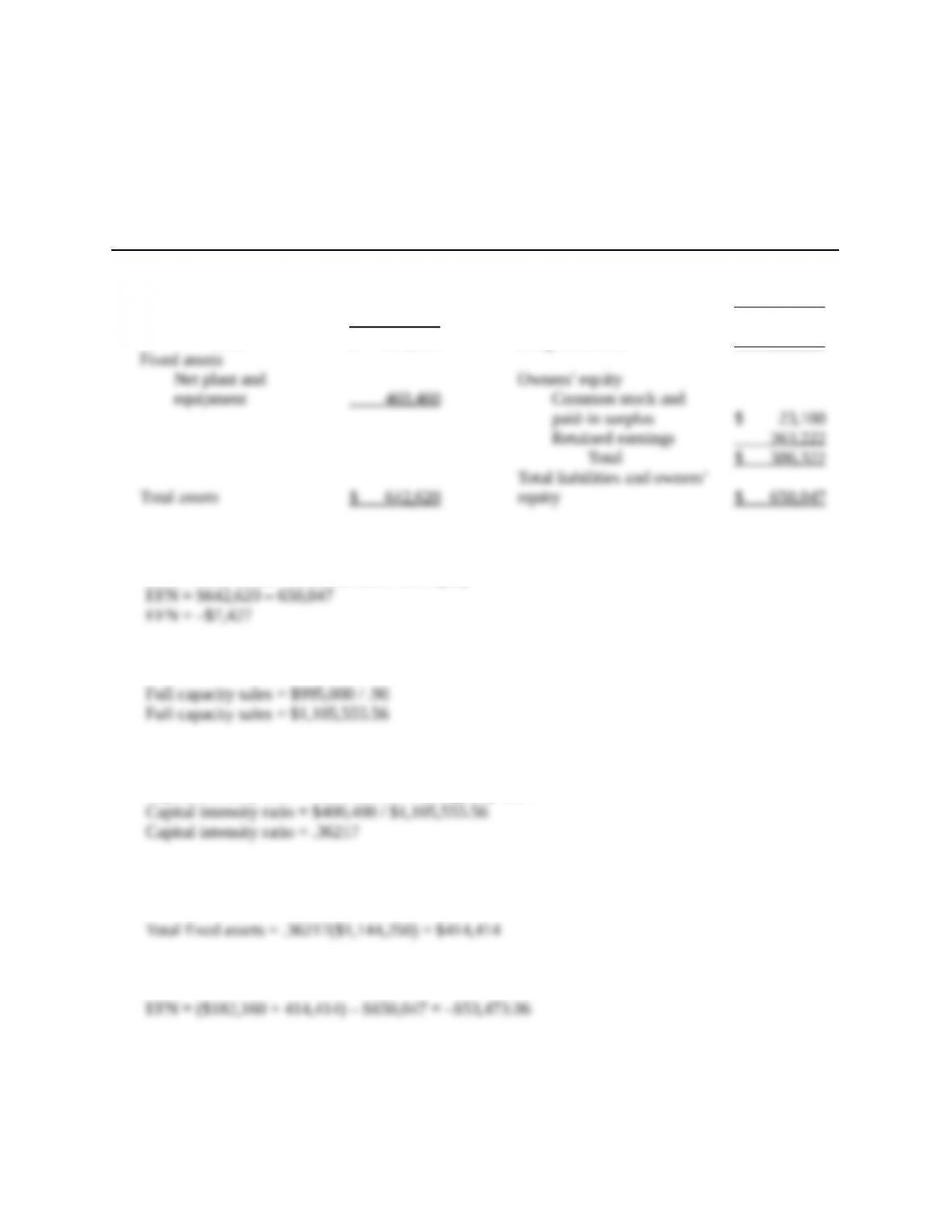The pro forma balance sheet will look like this:
MOOSE TOURS INC.
Pro Forma Balance Sheet
Assets Liabilities and Owners’ Equity
Current assets Current liabilities
Cash \$ 31,625 Accounts payable \$ 82,225
Accounts receivable 54,395 Notes payable 9,900
Inventory 96,140 Total \$ 92,125
Total \$ 182,160 Long-term debt 171,600
So, the EFN is:
EFN = Total assets – Total liabilities and equity
20. First, we need to calculate full capacity sales, which is:
The capital intensity ratio at full capacity sales is:
Capital intensity ratio = Fixed assets / Full capacity sales
The fixed assets required at full capacity sales is the capital intensity ratio times the projected sales
level:
So, EFN is:
Education.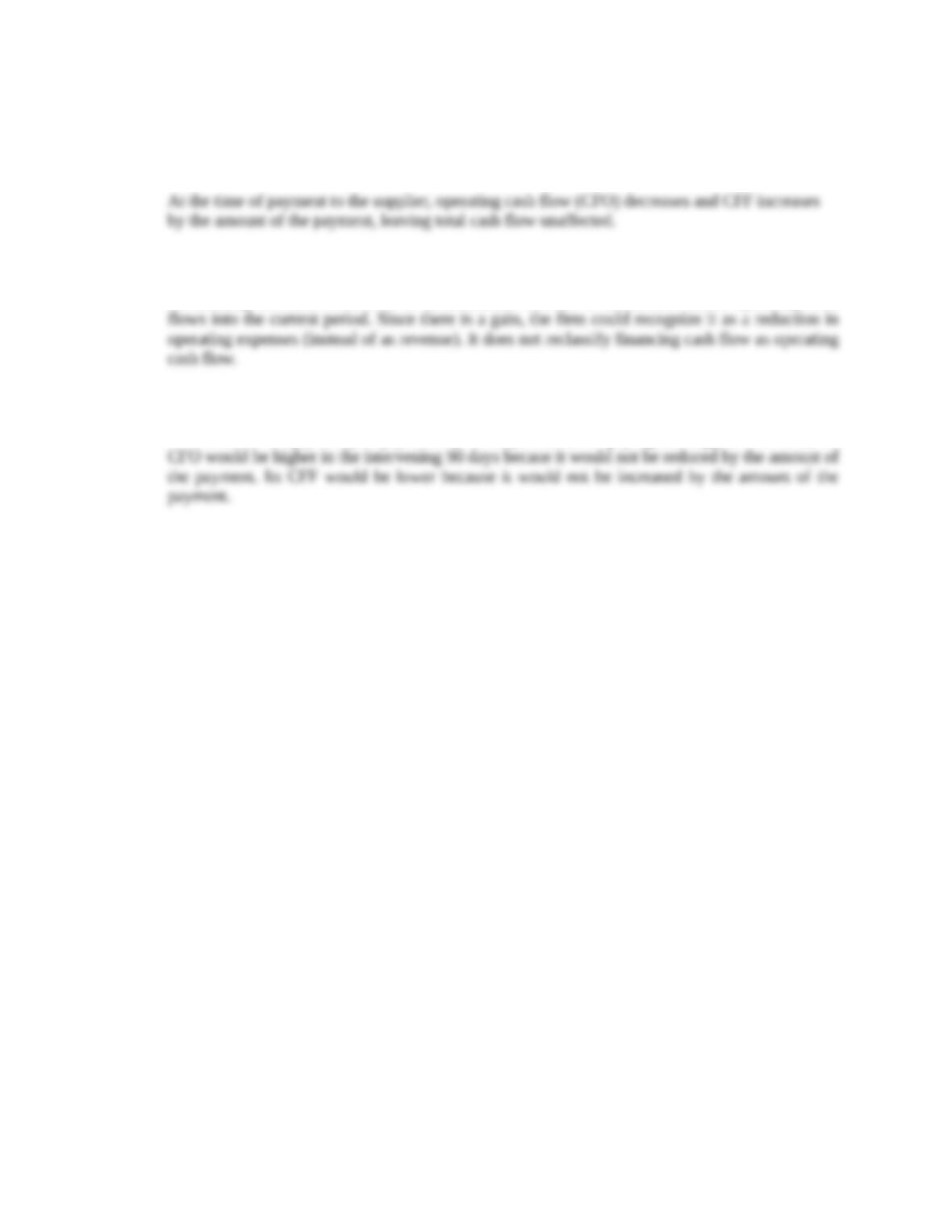CFA Exam Review by Kaplan Schweser
1. a
2. b
Securitizing accounts receivable is unsustainable because it accelerates future operating cash
3. a
If VirtualCon simply delayed payment by 90 days instead of paying the suppliers on time, its